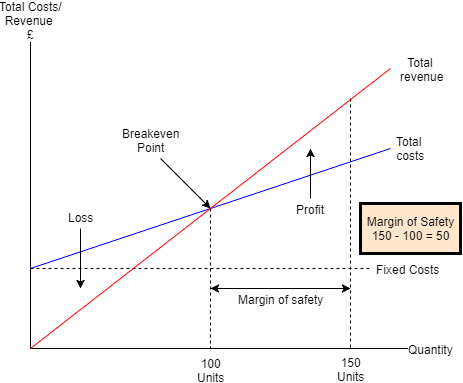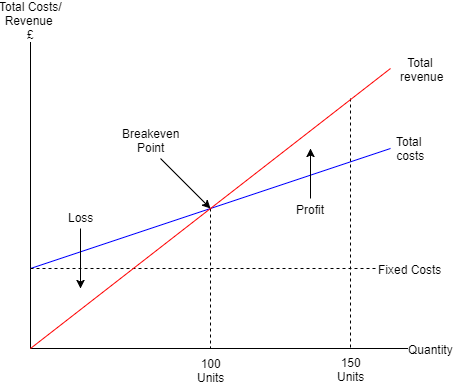Posted on

# 2.2.3 Break-even

A) Contribution

Contribution – The profit made on individual products.

Contribution = Selling price – Variable cost per unit.

B) Break-even point

Break-even – The point at which the business is making neither a profit nor a loss.

Break-even point = Total fixed costs + Total variable costs = Total revenue (The point at which Total costs = Total revenue)

C) Using contribution to calculate the break-even point

Break-even Total fixed costs/Contribution (Contribution already takes into account variable costs and therefore total variable costs is not included in the equation) – This shows the number of goods that need to be sold in order to reach the break-even point.

D) Margin of safetyMargin of safety – The difference between the actual level of output and the level of output needed to break even. This shows how much output can fall before the business reverts back to the break-even level of output. For example, if the break-even level of output was 2000 goods but the business’s actual level of output was 2500 goods then the margin of safety for the business would be 500 goods.

E) Interpretation of break-even chartsF) Limitations of break-even analysis

Assumes all output is sold – The break-even point assumes that all the goods made by the business will be sold.

Assumes prices are the same – The break-even point also assumes that the prices of the business’s products are all the same. However, businesses often have goods that are sold at different prices thus making it hard to work out the break-even point.

Changing variable costs – The variable costs of the business often change on a regular basis thus making it difficult for the business to use break-even as it would have to be updated regularly as variable costs change.